## 《scikit-learn》KMeans

2021/2/13 18:19:01 文章标签: 测试文章如有侵权请发送至邮箱809451989@qq.com投诉后文章立即删除

``````from sklearn.datasets import make_blobs
import matplotlib.pyplot as plt

# 自己创造一个数据集，
# 自己创造一个，一千个样本的数据，每个数据是2个特征，一共有4个中心
x, y = make_blobs(n_samples=1000, n_features=2, centers=4, random_state=54)
print(x.shape)
print(y.shape)
print(y[0:8])  # 返回的y呢就是以0为开始整数。

fig, ax1 = plt.subplots(1)  # 生成子图的数量，第一个是画布，第二个是对象
ax1.scatter(x[:, 0], x[:, 1],
marker='o',  # 点形状
s=8)  # 点大小
plt.title('original plain samples')
plt.show()

# 如果我们想看看点的分布的话，也可以画出来的，只是这正是我们聚类偶有做的事情啊。
# color = ['red', 'black', 'green', 'blue']
# fig, ax1 = plt.subplots(1)  # 生成子图的数量，第一个是画布，第二个是对象
# for i in range(4):
#     ax1.scatter(x[y == i, 0], x[y == i, 1],  # 使用布尔索引
#                 marker='o',  # 点形状
#                 s=8,  # 点大小
#                 c=color[i])  # 设置点颜色
#
# plt.title('original classified samples')
# plt.show()
``````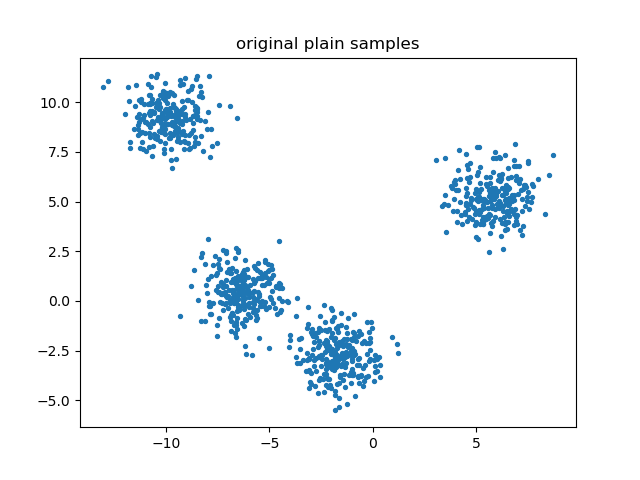``````# 开始聚类操作下
from sklearn.cluster import KMeans

n_clusters = 4
cluster = KMeans(n_clusters=n_clusters, random_state=9)
cluster = cluster.fit(x)  # 训练

pred = cluster.labels_
print(pred[0:8])  # 每个样本对应的结果
print(cluster.cluster_centers_)  # 返回得到的几个质心
print(cluster.inertia_)  # 总距离（所有点到各自质心的距离）的平方和，不是合适的模型评估指标

y_pred = cluster.fit_predict(x)
print(y_pred[0:8])  # 每个样本对应的结果

# 具体效果咋样，我们可视化一下看看
color = ['red', 'black', 'green', 'blue']
fig, ax1 = plt.subplots(1)  # 生成子图的数量，第一个是画布，第二个是对象
for i in range(4):
ax1.scatter(x[y_pred == i, 0], x[y_pred == i, 1],  # 使用布尔索引
marker='o',  # 点形状
s=8,  # 点大小
c=color[i])  # 设置点颜色
# 单独把质心画出来
ax1.scatter(cluster.cluster_centers_[:, 0], cluster.cluster_centers_[:, 1],  # 使用布尔索引
marker='x',  # 点形状
s=15,  # 点大小
c='yellow')  # 设置点颜色

plt.title('the result after KMeans')
plt.show()
``````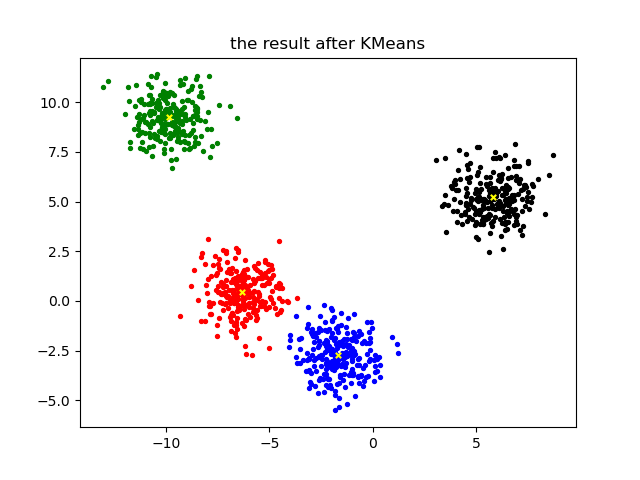1：我们使用轮廓系数来衡量，好的聚类算法的表现是，簇内的是很相似很近的，簇外就是越差异越远越好。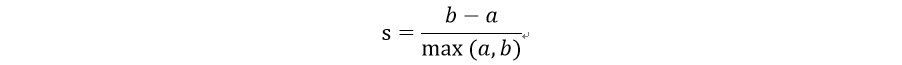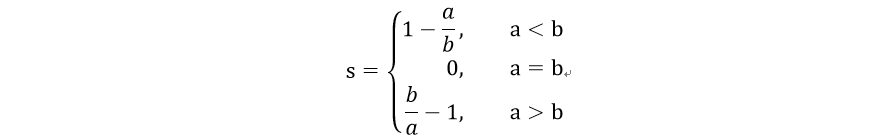``````# 衡量模型好坏的指标
from sklearn.metrics import silhouette_score
from sklearn.metrics import silhouette_samples

# 经过上面的计算，我们有了原始特征矩阵数据x,y，以及我们预测的y_pred

print(silhouette_score(x, y_pred))  # 计算轮廓系数，第一个是特征矩阵（样本坐标），第二个是聚类的结果
print(silhouette_score(x, cluster.labels_))  # 计算轮廓系数

silhouette_results = silhouette_samples(x, y_pred)
print(silhouette_results.shape)  # 展示每个样本的轮廓系数
print(silhouette_results.mean())  # 展示每个样本的轮廓系数综合后的平均值，就是silhouette_score的值。
``````

2：Calinski-Harabaz指数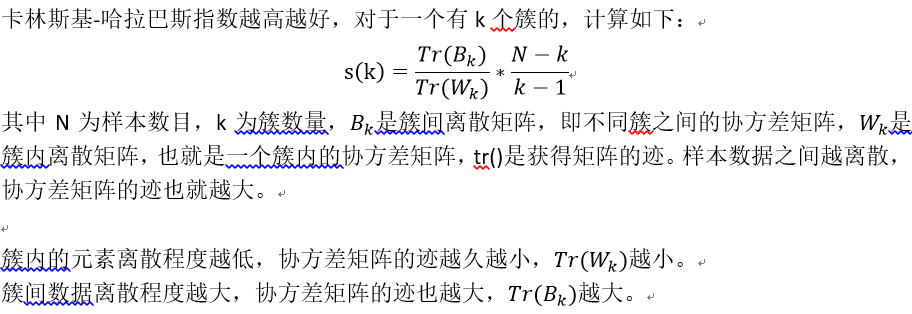``````from sklearn.metrics import calinski_harabasz_score

print(calinski_harabasz_score(x, y_pred))
print(calinski_harabasz_score(x, cluster.labels_))
``````

KMeans的第一步就是初始化质心，随机放置的效果也会不一样，最后会直接影响效果的，也会影响模型运行时间。

random_state就是质心随机初始化的种子，指定了的话，控制每次初始化质心都在相同的位置。

max_iter：可以让迭代提前终止，最大的迭代次数。
tol：如过两次迭代之间Inertial下降的量小于该值就结束掉迭代，基本已经不会有大变化了。它是浮点数，默认是1e-4。

KMeans的一个应用就是将非结构化数据（图像声音等）进行矢量量化，非结构化数据往往占用比较大的存储空间，数据量大，运算缓慢，我们希望在保证数据质量的情况下，尽可能减少数据的大小，简化结构化数据的结构。

``````from sklearn.datasets import load_sample_image  # 导入图片数据的类

from sklearn.cluster import KMeans
from sklearn.utils import shuffle  # 洗牌，打乱数据顺序的函数。

import matplotlib.pyplot as plt
import numpy as np
import pandas as pd

# 加载图片来试一试
print(china.dtype)  # 数组类型是uint8，0~255
print(china.shape)  # (427, 640, 3)，看来是RGB类型的数据。长度是427，宽度是640。RGB三通道

# 我们调整下数据形状
newimage = china.reshape(-1, 3)
print(newimage.shape)  # (273280, 3)，一张图片全部行程了一列，序列化了。RGB三通道也就是一个像素是三个值，一个像素3个特征。

uniquePiexls = pd.DataFrame(newimage).drop_duplicates()
print(uniquePiexls.shape)  # 哎呀(96615, 3)，说明重复度很大嘛。

# 图片的数据查看了，来看看效果。
plt.figure(figsize=(15, 15))
plt.title('original image')
plt.imshow(china)
plt.show()

# 加入哈，我们压缩到64个簇，计算每个原始样本到质心们的距离，找出最新的质心，然后用质心的数据来替换原始数据的值。我们可以观察原始数据的效果。
n_clusters = 64

# 预处理一下，所有的数字都压缩到了[0,1]之间
china = np.array(china, dtype=np.float64) / china.max()
print(china.shape)
print((china > 1).sum())
print((china < 0).sum())

image_array = np.reshape(china, (-1, 3))
print(image_array.shape)

# 上模型
# 训练方式一：太慢了
# kmeans = KMeans(n_clusters=n_clusters, random_state=12).fit(image_array)
# print(kmeans.cluster_centers_.shape)

# 训练方式二：先使用部分数据找出质心，再使用这些质心预测所有点，这样快多了啊
image_array_samples = shuffle(image_array)[:5000]  # 比如先使用5000个像素样本来搞事情
kmeans = KMeans(n_clusters=n_clusters, random_state=12).fit(image_array_samples)
print(kmeans.cluster_centers_.shape)

# 然后预测出所有的像素点的值
labels = kmeans.predict(image_array)  # 生成了簇的质心的簇索引。
print(labels.shape)

# 使用质心来替换所有样本啊

image_kmeans = kmeans.cluster_centers_[labels]
print(image_kmeans.shape)
image_kmeans = image_kmeans * china.max()  # 恢复到0-255
image_kmeans = image_kmeans.reshape(china.shape, china.shape, china.shape)  # 转换到三维。此时图像只有64个像素重复了。

plt.figure(figsize=(15, 15))
plt.title('after kmeans')
plt.imshow(image_kmeans)
plt.show()

# 至此我们把图像进行了压缩，我们只需要存储labels的序列，以及所有的质心，kmeans.cluster_centers_即可保存原始的图像。
``````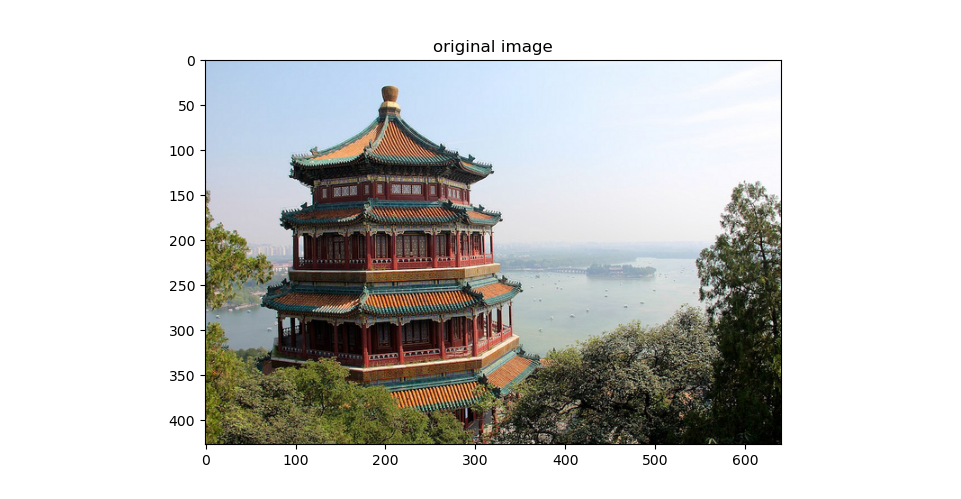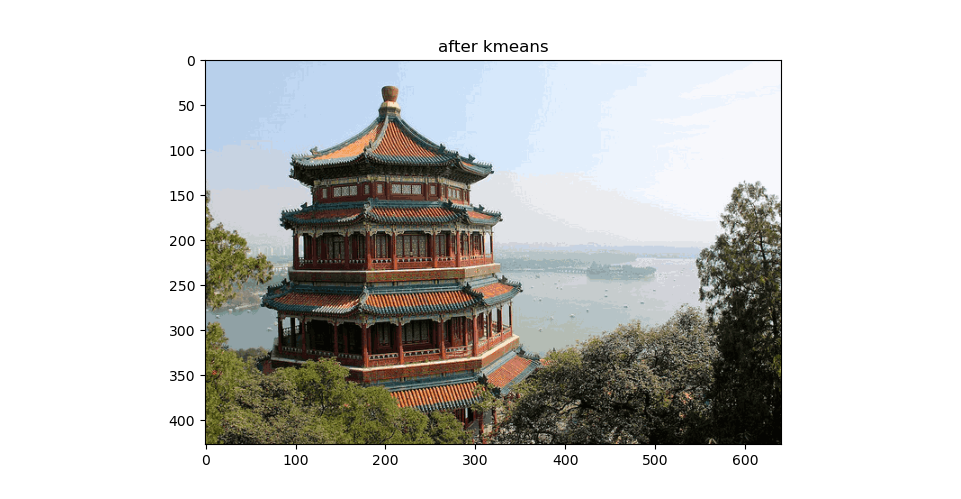暂无相关的数据...